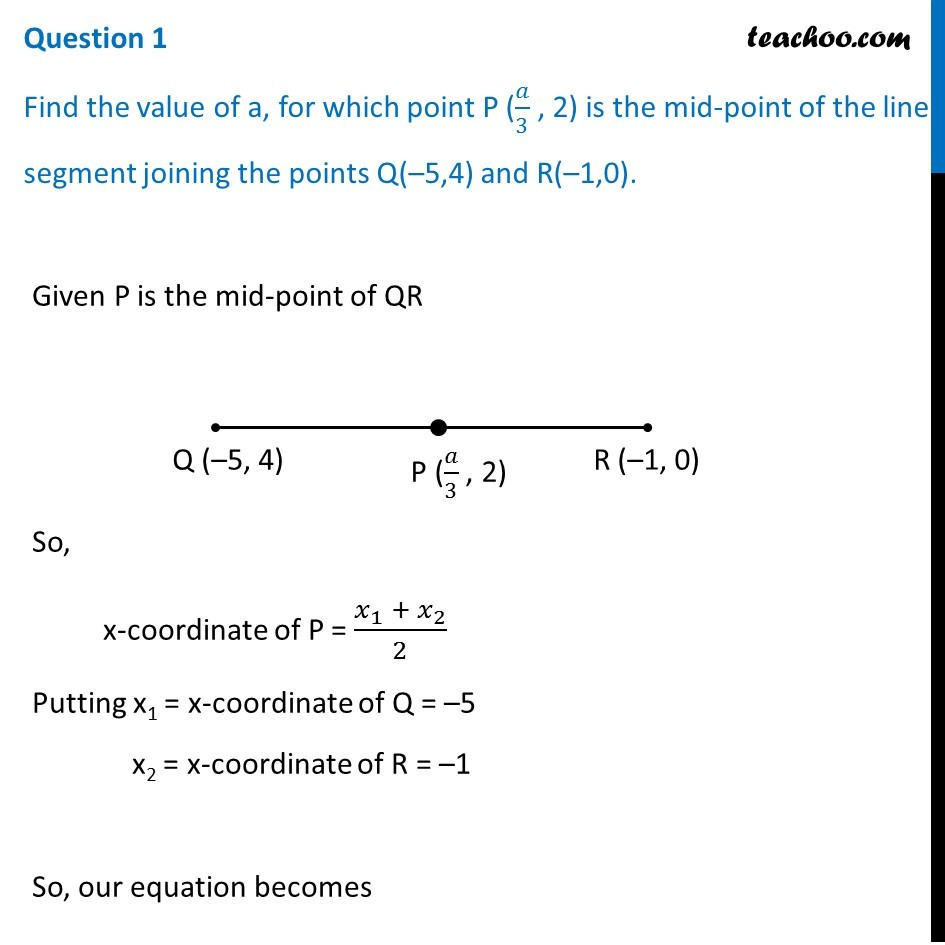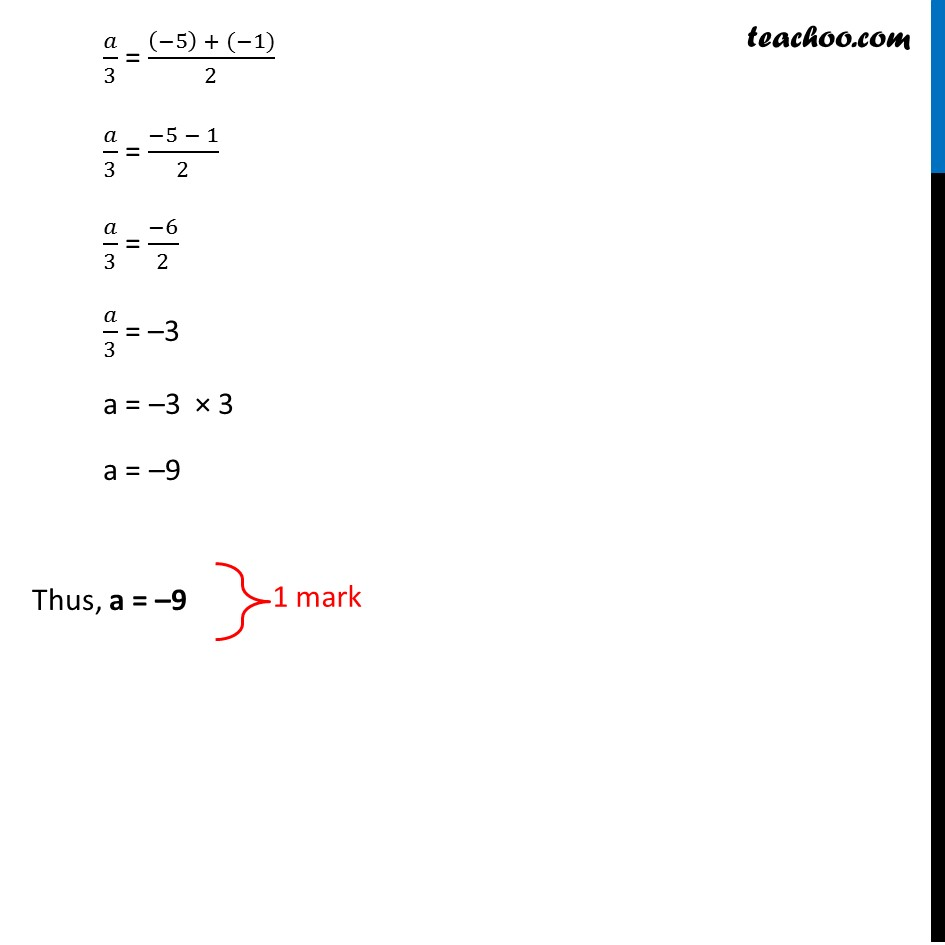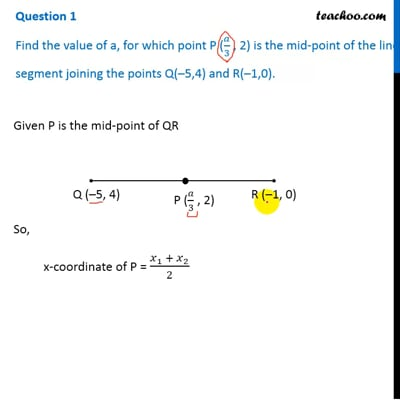CBSE Class 10 Sample Paper for 2019 Boards

Class 10
Solutions of Sample Papers for Class 10 Boards

Question 1 of CBSE Maths Sample Paper for 2019 Boards

Find the value of a, for which point P (a/3 , 2) is the mid-point of the line segment joining the points Q(–5,4) and R(–1,0).This video is only available for Teachoo black users

Introducing your new favourite teacher - Teachoo Black, at only ₹83 per month

### Transcript

Question 1 Find the value of a, for which point P (𝑎/3 , 2) is the mid-point of the line segment joining the points Q(–5,4) and R(–1,0). Given P is the mid-point of QR So, x-coordinate of P = (𝑥_1 + 𝑥_2)/2 Putting x1 = x-coordinate of Q = –5 x2 = x-coordinate of R = –1 So, our equation becomes 𝑎/3 = ((−5) + (−1))/2 𝑎/3 = (−5 − 1)/2 𝑎/3 = (−6)/2 𝑎/3 = –3 a = –3 × 3 a = –9 Thus, a = –9